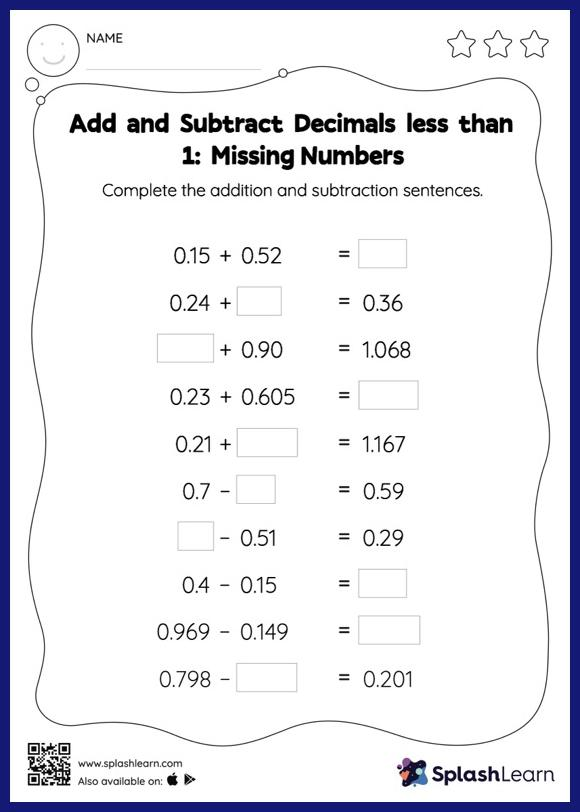# Add and Subtract Decimals less than 1: Missing Numbers Worksheet

Home > Add and Subtract Decimals less than 1: Missing NumbersThis add and subtract decimals less than 1 worksheet has a set of problems curated to ensure that your child becomes more proficient in addition and subtraction. Students align the decimal points and use zero as space holders to add and subtract decimals. Then they use the relationship between addition and subtraction to find the missing number in add and subtract decimals less than 1 worksheet.